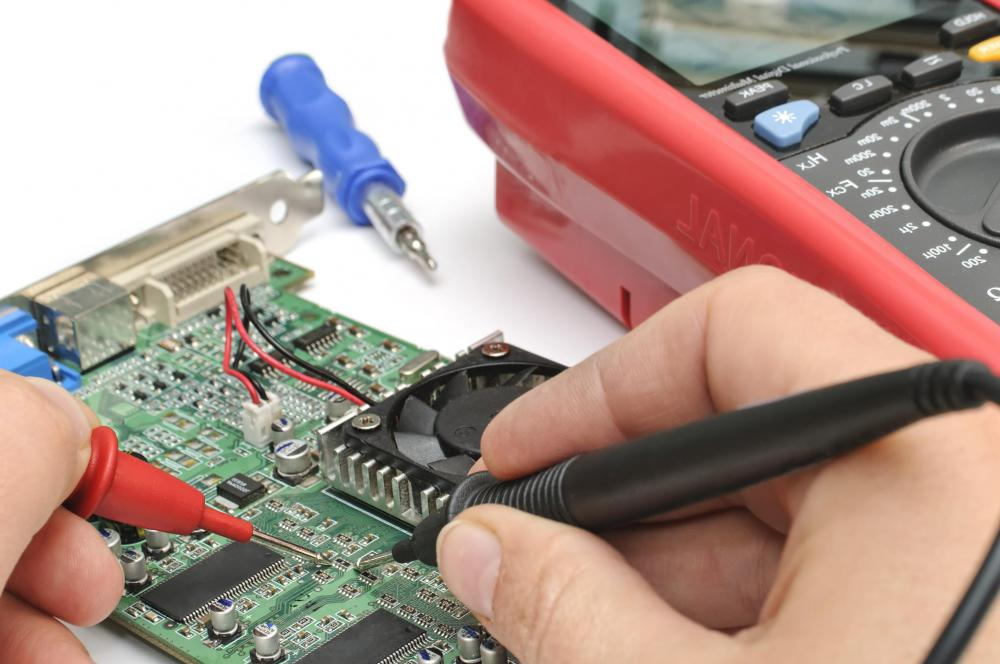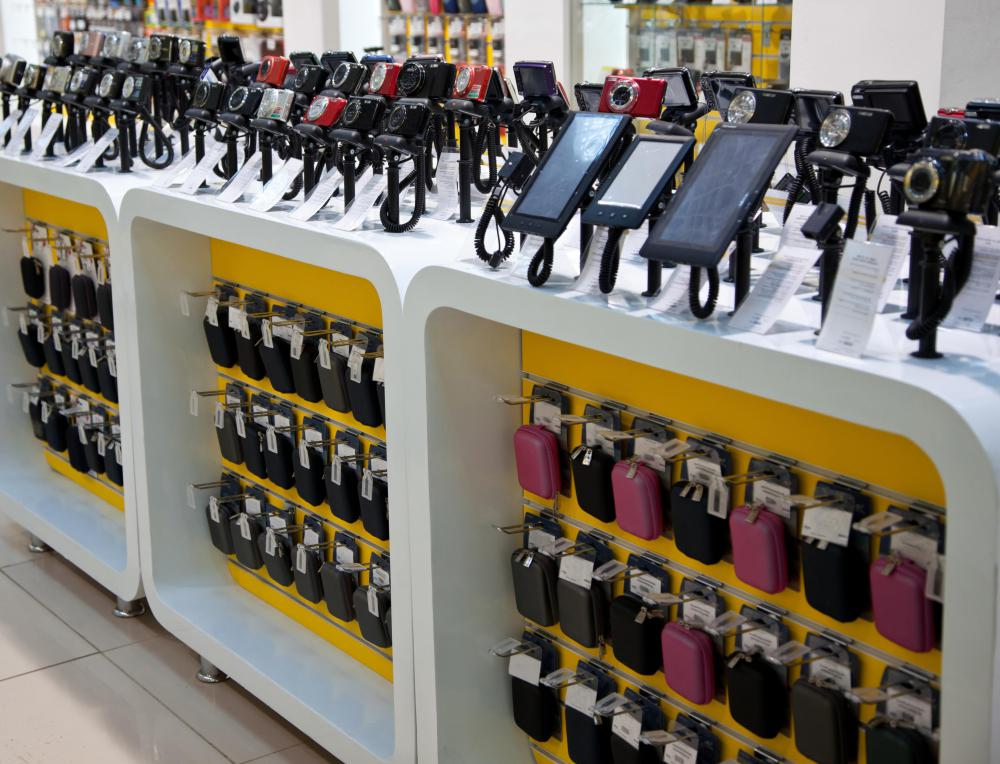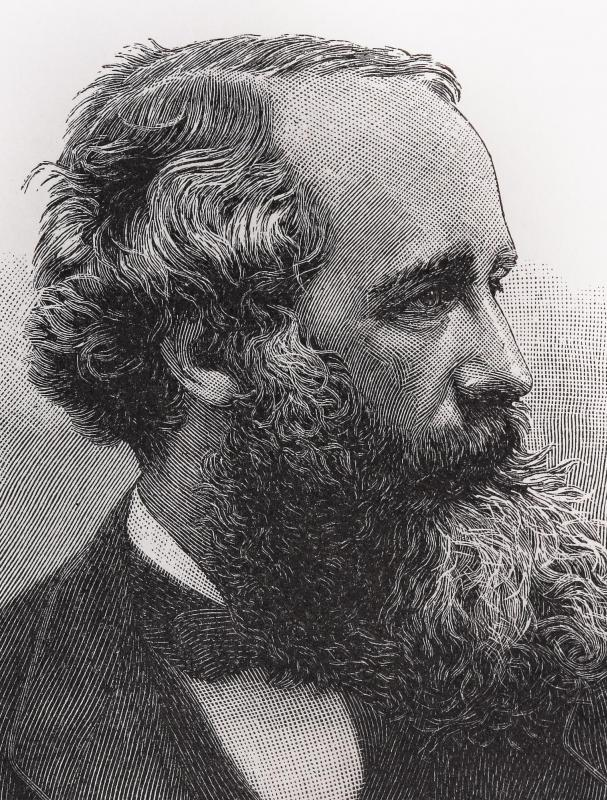# What is Capacitance? (with pictures)

Jason C. Chavis
Jason C. ChavisCapacitance is measured in farads.

The ability to hold an electric charge is known as voltage capacitance. It is found most commonly in electromagnetic fields, which exert some sort of physical force on particles. The force causes the particles to move, which results in an electric charge.

Electronics use capacitance as a basic component. Devices known as semiconductors help the flow of electrons through conductors made of nonmetallic materials. They work with other electronic devices, most notably capacitors, to make that flow work to power and control a large amount of components.Capacitance is a basic component of electronics.

Capacitors are the main component that harnesses the electric charge. These are essentially a pair of conductors that contain movable electric charge separated by a dielectric or insulator. In order for an electric field to be present inside the insulator, a difference between the voltage of each conductor must be present — this is known as the potential difference. As the energy is stored, a mechanical force is produced between the conductors. This is most common between flat and narrowly separated conductors.James Clerk Maxwell conceived of capacitance in 1861.

When two capacitors are placed close together for a period of time they create an effect known as “stray capacitance.” This means that the electric charge loses some of its signal and begins to leak within the isolated currents. The effect is detrimental for the proper function of high frequency currents.

Self-capacitance must also be used within a number of electrical devices. This occurs by increasing the electrical charge by the amount that is needed to raise the potential by one volt. One way to allow this to happen is by placing a hollow conducting sphere between the conductor, which makes the capacitor regulate itself in regards to electrical charge.

Capacitance is generally considered the inverse of inductance, the concept of resisting a change in current flow. Both phenomena can be measured by substituting the voltage and current number within each equation with the opposite measurement. In the same way, an inductor will offset the function of a capacitor.

The holding of an electric charge is measured in farads. This is the amount of electric charge potential that can change one volt within a capacitor. It also measures the amount of electric charge that can be transported in a single second by a steady current.

The concept of capacitance was first conceived in 1861 by James Clerk Maxwell. He invented the concept of displacement current, which is the rate of change within the electromagnetic field. Maxwell understood the concepts of insulators and how electricity flowed through them, as well as how electromotive force would produce a state of polarization in its parts.

## You might also Like## Discuss this Article

Forgot password?
###### Register:
•Capacitance is measured in farads.
•Capacitance is a basic component of electronics.
•James Clerk Maxwell conceived of capacitance in 1861.